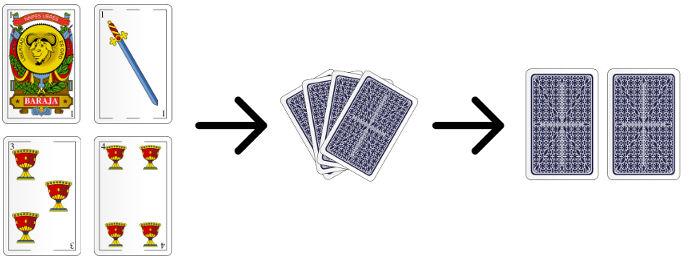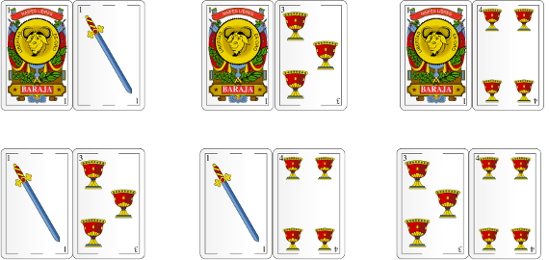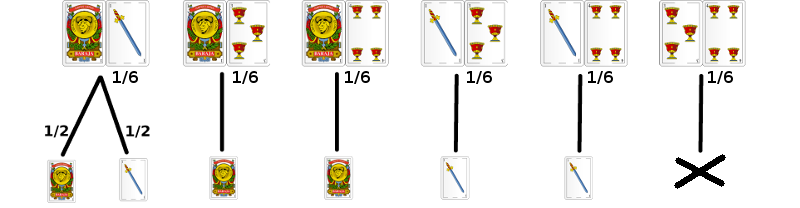## 𓃰 The Diligent Developer

25 Jul, 2016

A few days ago my former programming teammate Ivan Geffner presented me a curious mathematical paradox that I will now explain. This problem appears in the book Reasoning about uncertainty by Joseph Halpern, a professor at Cornell University, where Ivan is doing his doctorate. Let's see what it is.

### The game

A friend of ours presents us a game using four cards (from the Spanish deck): the ace of golds, ace of spades, the three and four of cups. Repeatedly, our friend shuffles these four cards and we pick two of them at random:We randomly choose two cards from these four.

Then he offers a deal:

—Would you bet 1€ to having both aces? If you do have them, I will give you 4€.

As we are reasonable people, we will make some small probability calculations before entering the game. We note that there are six possible combinations of two cards, all equally probable:The six possible choices of two cards

So, ultimately, we will win only 1 time in 6, and thus lose 6€ in order to win only 4€. So we tell our friend: I will not play!

Our friend keeps shuffling the four cards and handing us two of them. He looks at the cards we have discarded, and to make the game more attractive to us, he says:

Proposal #1. —I can see you at least have an ace, do you want to play now?

Of the six initial combinations, we can dismiss the case where we have the three and the four, because we know that at least we have one ace. Then there are five possibilities:In 1 case out of 5 we have two aces, so we have to spend 5€ to earn 4€. The answer is the same as before: I do not play!

Making a great effort, our friend keeps dealing and trying to offer us even better deals, like this one:

Proposal #2. —Now you have the ace of golds, do you want to play?

Because we know that we have the ace of golds, there are only three possible combinations:So every 3 times we bet, we will win once, so we will earn 4€ for every 3€ we bet! Then we tell our friend: I want to play!

Similarly, after dealing again, the friend suggests:

Proposal #3. —Now you have the ace of spades, wanna play?

This case is equivalent to the one before, so we will also play! Having the ace of spades, there are only three possible choices:Finally, our friend reveals the paradox:

—When I tell you that you have the ace of golds or the ace of spades you decide to play. However, when I tell you that you have an ace (without specifying which one of them), you don't want to play. But it is obvious that if you have an ace you will have either the ace of golds or the ace of spades!

What is the explanation of this paradox?

The truth is that we were a little hasty when we bet our savings to a game without a thorough understanding of its probabilities.

The key lies in the information our friend gives us, especially when we have two aces. If we have two aces, which one does he tell us that we have: the ace of golds or the ace of spades? Suppose he randomly says one of them and let's calculate the odds again.

When our friend tells us that we have the ace of golds, there are three possibilities:

• We have the ace of golds and the three of cups, with probability $\frac16$.
• We have the ace of golds and the four of cups, with probability $\frac16$.
• We have both aces (probability $\frac16$), and our friend chose to tell us that we have the ace of golds (probability $\frac12$). The probability is $\frac16\cdot\frac12=\frac1{12}$.Probabilities of all possible outcomes of the game

Thus the probability of having both aces when our friend tells us that we have the ace of golds is:

$$P=\frac{\frac1{12}}{\frac16 + \frac16 + \frac1{12}} = \frac{\frac1{12}}{\frac5{12}} = \frac15$$

In short, we'd better not play in any of the cases.

To understand this paradox we have had to think accurately about how the game develops, instead of calculating some isolated probabilities that do not match the real problem. Joseph Halpern presents in his book a mathematical framework with different measures of probability, conditioning, expectation, protocols, etc. and formalizes the concepts needed to tackle and solve these problems.